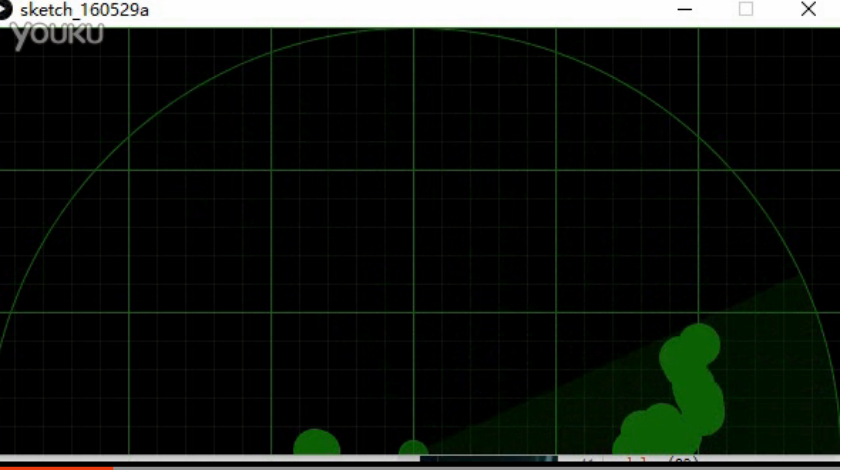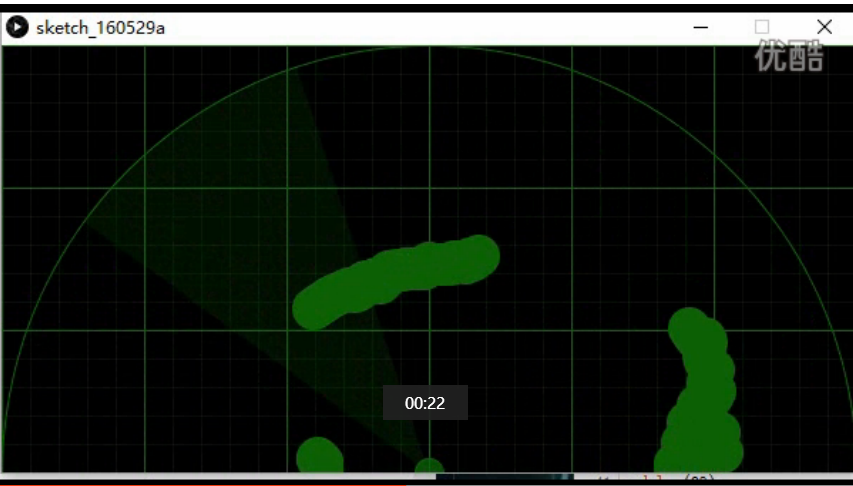# 使用Processing+Arduino写的类似雷达扫描效果arduino uno板子一块

processing 接受到串口的数据。解析出来角度和距离。 将这个数据。放入数组中的对应角度下标值为这个角度的距离。

processing的效果大概是这样的。 Arduino+Processing雷达扫描效果

arduino程序方面。

//当前角度
int mAngleNum = 0;
//当前是正向旋转还是反向旋转
char mFront = 0;

//设置舵机当前的角度
mServo.write(180 - mAngleNum);

if( mFront == 0 )
{
mAngleNum ++;
if( mAngleNum > 180 )
{
mFront = 1;
}
}
else
{
mAngleNum --;
if( mAngleNum < 0 )
{
mFront = 0;
}
}


//当前距离
int mDistance = 0;
//超声波测距引脚
const int mTrigPin = 2;
const int mEchoPin = 3;

pinMode(mTrigPin, OUTPUT);
// 要检测引脚上输入的脉冲宽度，需要先设置为输入状态
pinMode(mEchoPin, INPUT);

// 产生一个10us的高脉冲
digitalWrite(mTrigPin, LOW);
delayMicroseconds(2);
digitalWrite(mTrigPin, HIGH);
delayMicroseconds(10);
digitalWrite(mTrigPin, LOW);

mDistance = pulseIn(mEchoPin, HIGH) / 58.0; //将回波时间换算成cm


if ( myPort.available() > 0) {
}

int mWidth = 600;
int mHeight = 300;

for( int i = 0; i < 181; i ++ )
{
int a, b, distance;
distance = a = b = mDistanceArr[i];

if( i - 1 >= 0 )
a = mDistanceArr[ i - 1 ];
if( i + 1 <= 180 )
b = mDistanceArr[ i + 1 ];
if( distance < 5 || distance > mMaxDistance ) continue;
if( a < 5 || a > mMaxDistance ) a = distance;
if( b < 5 || b > mMaxDistance ) b = distance;

distance = (a + b + distance) / 3;

if( distance < 5 || distance >= mMaxDistance ) continue;
//distance = 60 angle = 45;
//mWidth / 2;
float scale = mWidthHalf / mMaxDistance;

int tmpDistance = (int)(distance * scale);

int px = (int)(mWidthHalf - tmpDistance * cos(i * PI/180));
int yy = (int)(tmpDistance * sin(i * PI/180));
int py = 300 - yy;
println("scale:" + scale + " angle:" + i + " sin():" + sin(i * PI/180) + " distance:" + distance + " tmpDistance:" + tmpDistance + " x:" + px + " yy:" + yy + "  py:" + py);

ellipse(px, py, 30, 30);
}

10-29
09-0729808-071万+
02-241191
11-08
12-29
03-29
12-101534